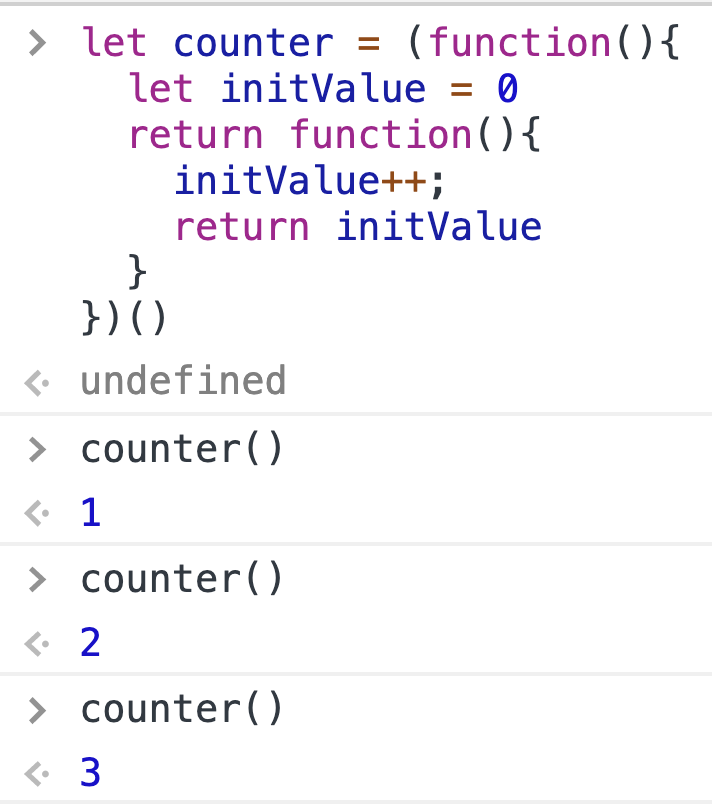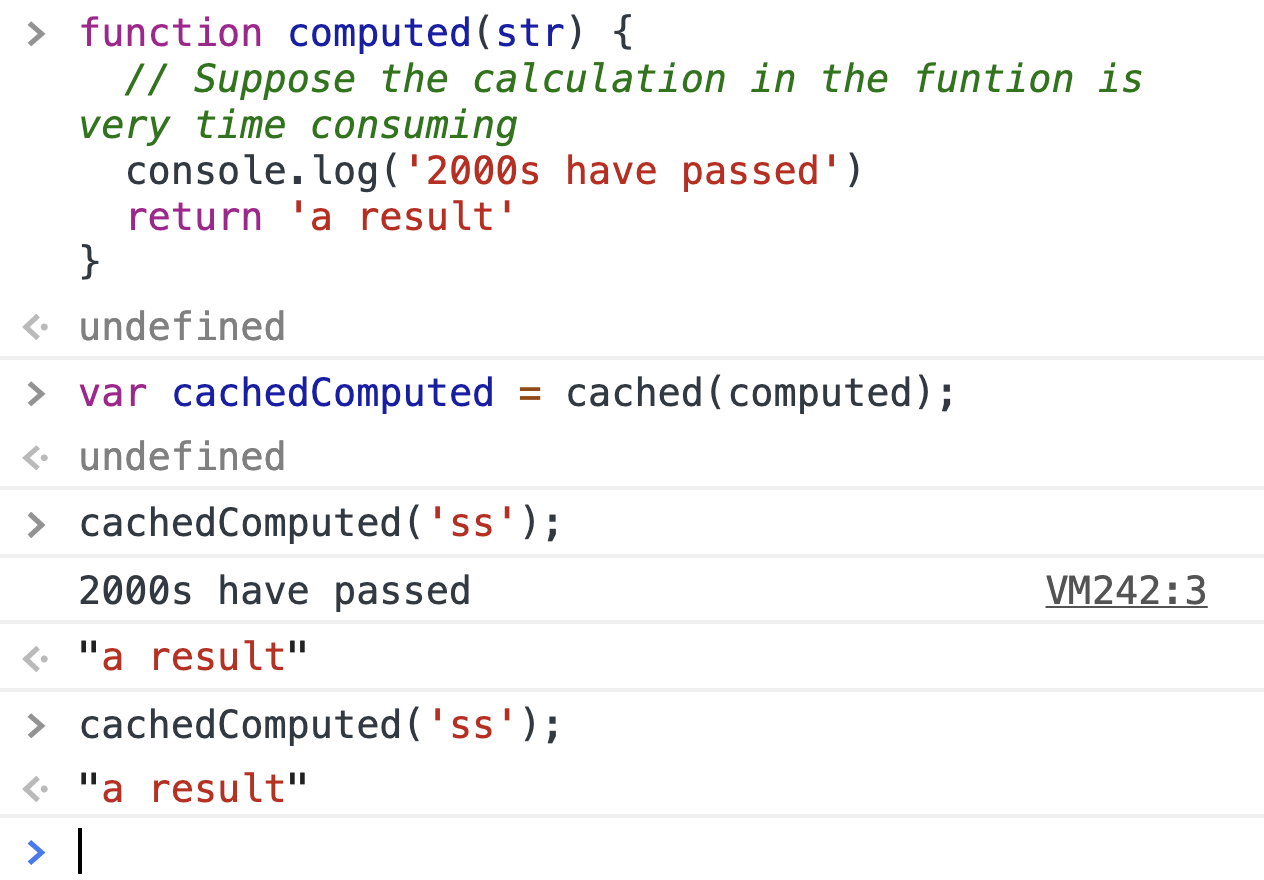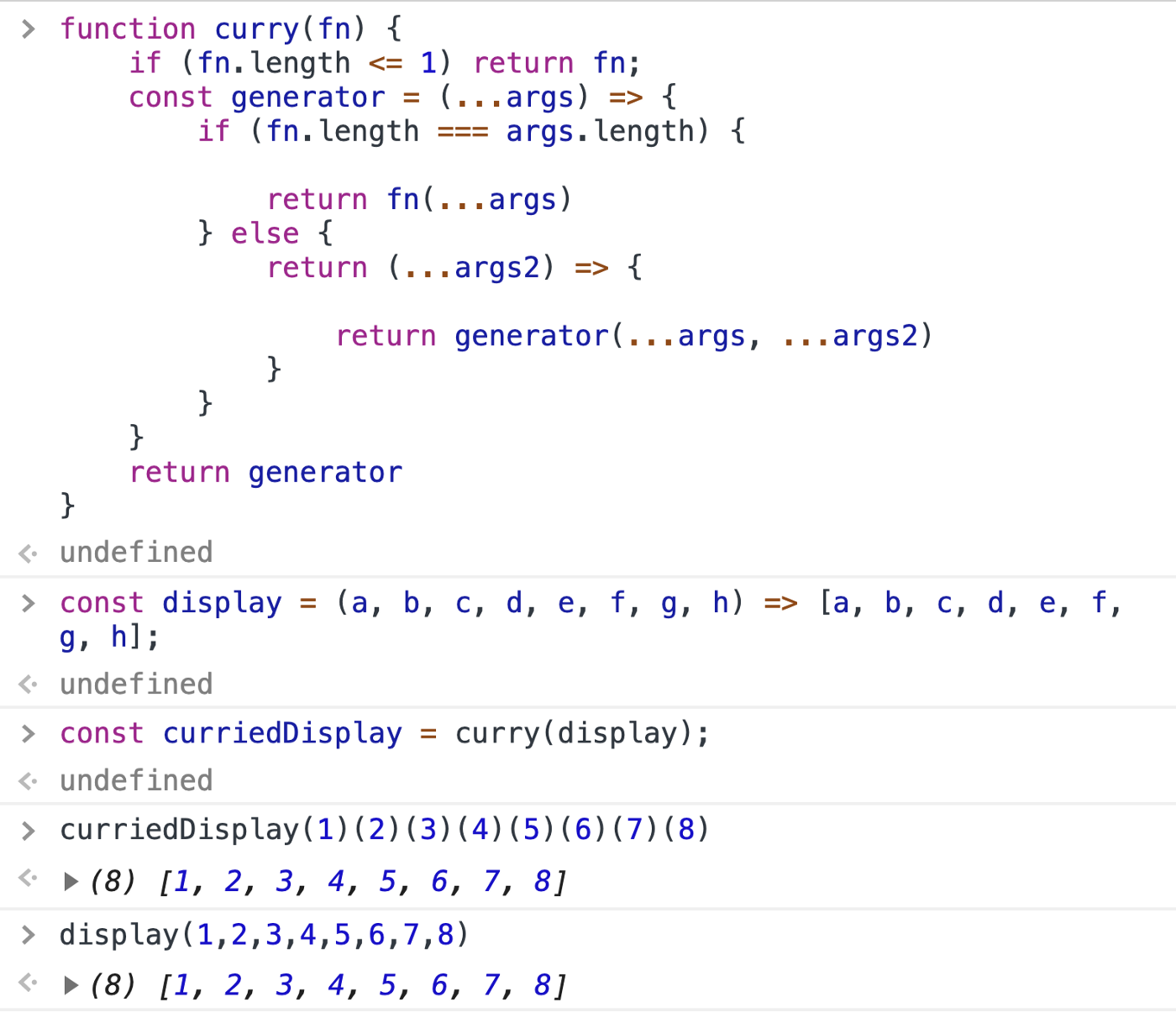# View:JavaScript函数的秘密

Posted by Dan on November 4, 2020

View:《Functions in JavaScript Have More Secrets Than You Think》

• 纯函数
• 高阶函数
• 函数缓存
• 懒函数
• 函数柯里化
• 函数组件

## 纯函数（Pure Function）

• 如果传相同的参数，总是返回相同的结果；
• 在执行函数时，没有副作用（side effect）

1
2
3
function circleArea(radius){
return radius * radius * 3.14
}


1
2
3
4
5
6
7
let counter = (function(){
let initValue = 0
return function(){
initValue++;
return initValue
}
})()1
2
3
4
5
6
7
8
9
let femaleCounter = 0;
let maleCounter = 0;
function isMale(user){
if(user.sex = 'man'){
maleCounter++;
return true
}
return false
}


1. 纯函数要清晰的多，也更容易阅读。

1. 编译器可以对纯函数进行更多的优化。

1
2
3
for (int i = 0; i < 1000; i++){
console.log(fun(10));
}


1
2
3
4
let result = fun(10)
for (int i = 0; i < 1000; i++){
console.log(result);
}

1. 纯函数更容易测试

1
2
3
const incrementNumbers = function(numbers){
// ...
}


1
2
let list = [1, 2, 3, 4, 5];
assert.equals(incrementNumbers(list), [2, 3, 4, 5, 6])


## 高阶函数（Higher-Order Function）

• 将一个或多个函数作为参数
• 返回一个函数作为其结果

1
2
3
4
5
const arr1 = [1, 2, 3];
const arr2 = [];
for (let i = 0; i < arr1.length; i++) {
arr2.push(arr1[i] * 2);
}


The map(callback) method creates a new array populated with the results of calling a provided function on every element in the calling array.

1
2
3
4
5
const arr1 = [1, 2, 3];
const arr2 = arr1.map(function(item) {
return item * 2;
});
console.log(arr2);


map 函数是一个高阶函数。

## 函数缓存（Function Caching）

1
2
3
4
5
6
7
function computed(str) {
// Suppose the calculation in the funtion is very time consuming
console.log('2000s have passed')

// Suppose it is the result of the function
return 'a result'
}## 懒函数（Lazy Function）

1
2
3
4
5
6
7
8
9
let fooFirstExecutedDate = null;
function foo() {
if ( fooFirstExecutedDate != null) {
return fooFirstExecutedDate;
} else {
fooFirstExecutedDate = new Date()
return fooFirstExecutedDate;
}
}


1
2
3
4
5
6
7
var foo = function() {
var t = new Date();
foo = function() {
return t;
};
return foo();
}


1
2
3
4
5
6
7
8
function addEvent (type, el, fn) {
if (window.addEventListener) {
el.addEventListener(type, fn, false);
}
else if(window.attachEvent){
el.attachEvent('on' + type, fn);
}
}


1
2
3
4
5
6
7
8
9
10
11
12
function addEvent (type, el, fn) {
if (window.addEventListener) {
addEvent = function (type, el, fn) {
el.addEventListener(type, fn, false);
}
} else if(window.attachEvent){
addEvent = function (type, el, fn) {
el.attachEvent('on' + type, fn);
}
}
return addEvent(type, el, fn)
}


## 函数柯里化（Function Curring）

• 柯里化可以帮助你避免一次又一次地传递同一个变量
• 它有助于创建一个高阶函数，在事件处理方面非常有帮助
• 小件可以配置和轻松重复使用

1
2
3
function add(a,b,c){
return a + b + c;
}


1
2
3
add(1,2,3) --> 6
add(1,2) --> NaN
add(1,2,3,4) --> 6 //Extra parameters will be ignored.## 函数组合（Function Compose）

Input ‘bitfish’, return ‘HELLO, BITFISH’.

• 串联字符串
• 将字符串转换为大写

1
2
3
4
5
6
7
8
9
10
let toUpperCase = function(x) {
return x.toUpperCase();
};

let hello = function(x) {
return 'HELLO, ' + x;
};
let greet = function(x){
return hello(toUpperCase(x));
};


1
2
3
4
5
let compose = function(f,g) {
return function(x) {
return f(g(x));
};
};


1
2
let greet = compose(hello, toUpperCase);
greet('kevin');


1
2
3
4
5
6
7
8
9
10
function compose() {
var args = arguments;
var start = args.length - 1;
return function() {
var i = start;
var result = args[start].apply(this, arguments);
while (i--) result = args[i].call(this, result);
return result;
};
};